# Measuring Length to the Nearest Millimeter Online Quiz

Following quiz provides Multiple Choice Questions (MCQs) related to Measuring Length to the Nearest Millimeter. You will have to read all the given answers and click over the correct answer. If you are not sure about the answer then you can check the answer using Show Answer button. You can use Next Quiz button to check new set of questions in the quiz.Q 1 - Find the length of the following object to the nearest millimeter.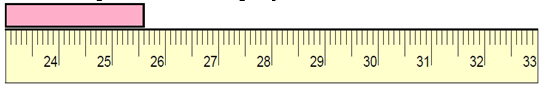### Explanation

Step 1:

The reading is closer to 256 mm than to 255 mm.

Step 2:

So, the length of the given object to the nearest millimeter is 256 mm.

Q 2 - Find the length of the following object to the nearest millimeter.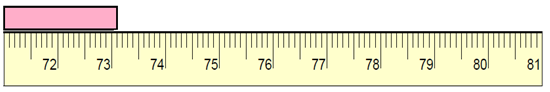### Explanation

Step 1:

The reading is closer to 731 mm than to 732 mm.

Step 2:

So, the length of the given object to the nearest millimeter is 731 mm.

Q 3 - Find the length of the following object to the nearest millimeter.### Explanation

Step 1:

The reading is closer to 462mm mm than to 463 mm.

Step 2:

So, the length of the given object to the nearest millimeter is 462 mm.

Q 4 - Find the length of the following object to the nearest millimeter.### Explanation

Step 1:

The reading is closer to 916 mm than to 915 mm.

Step 2:

From the reading on the ruler, the length of the given object to the nearest millimeter is 916 mm.

Q 5 - Find the length of the following object to the nearest millimeter.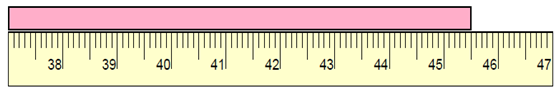### Explanation

Step 1:

The reading is closer to 455 mm than to 454 mm.

Step 2:

So, the length of the given object to the nearest millimeter is 455 mm.

Q 6 - Find the length of the following object to the nearest millimeter.### Explanation

Step 1:

The reading is closer to 307 mm than to 308 mm.

Step 2:

So, the length of the given object to the nearest millimeter is 307 mm.

Q 7 - Find the length of the following object to the nearest millimeter.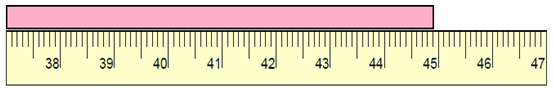### Explanation

Step 1:

The reading is closer to 449 mm than to 448 mm.

Step 2:

So, the length of the given object to the nearest millimeter is 449 mm.

Q 8 - Find the length of the following object to the nearest millimeter.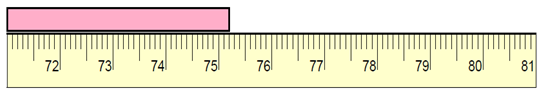### Explanation

Step 1:

The reading is closer to 752 mm than to 753 mm.

Step 2:

So, the length of the given object to the nearest millimeter is 752 mm.

Q 9 - Find the length of the following object to the nearest millimeter.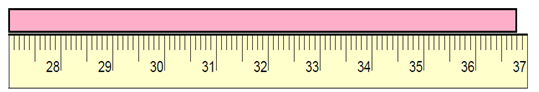### Explanation

Step 1:

The reading is closer to 368 mm than to 367 mm.

Step 2:

So, the length of the given object to the nearest millimeter is 368 mm.

Q 10 - Find the length of the following object to the nearest millimeter.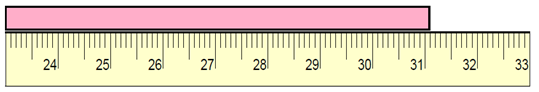### Explanation

Step 1:

The reading is closer to 311 mm than to 310 mm.

Step 2:

So, the length of the given object to the nearest millimeter is 311 mm.

measuring_length_to_the_nearest_millimeter.htm